# CT系统模型新模板设计的发现A Discovery of New Template Design of CT System Model

• 全文下载: PDF(3617KB)    PP.496-502   DOI: 10.12677/CSA.2018.84055
• 下载量: 308  浏览量: 563   科研立项经费支持

Computed Tomography (CT) technology has been widely used in the field of medicine and engineering. The shape of the template is crucial to the calibration of CT system parameters. In this pa-per, the ordinary single ellipse and small circle template are improved. We design three new templates. The receiving information is obtained by Radon transform and R-L filter firstly, and then their reconstruction images are acquired based on convolution back projection reconstruction algorithm. Furthermore, we make a quantitative assessment of the reconstruction effect by the sensitivity, specificity and Youden index. In addition, in order to investigate the anti-noise, the original template and the new templates are added to the Gauss noise of different strength, respectively. The experimental result shows that the new templates have better noise resistance than the original template. An interesting finding is that the template generated by geometric figure of a single reference frame with polygon outline is better than the template produced by multi reference frame geometry with the arc line for the reconstruction of CT.

1. 引言

2. 数据来源和模型假设

3. 卷积反投影重构图像

${h}_{R-L}\left(nd\right)=\left\{\begin{array}{ll}1/\left(4{d}^{2}\right)\hfill & n=0;\hfill \\ 0\hfill & n为偶数;\hfill \\ -1/\left({n}^{2}{\text{π}}^{2}{d}^{2}\right)\hfill & n为奇数.\hfill \end{array}$ (1)

$\begin{array}{c}\stackrel{˜}{p}\left(n,m\right)=p\left(n,m\right)×h\left(n\right)\\ =\underset{l=-{N}_{i}}{\overset{{N}_{i}}{\sum }}p\left(n-l,m\right)h\left(l\right)\text{\hspace{0.17em}}\text{\hspace{0.17em}}\text{\hspace{0.17em}}\text{\hspace{0.17em}}\text{\hspace{0.17em}}\text{\hspace{0.17em}}\text{\hspace{0.17em}}n=0,1,2,\cdots ,255\end{array}$ (2)

$\begin{array}{c}\stackrel{˜}{p}\left({n}_{0}+\delta \right)=\stackrel{˜}{p}\left({n}_{0}\right)+\delta \left[\stackrel{˜}{p}\left({n}_{0}+1\right)-\stackrel{˜}{p}\left({n}_{0}\right)\right]\\ =\left(1-\delta \right)\stackrel{˜}{p}\left({n}_{0}\right)+\delta \stackrel{˜}{p}\left({n}_{0}+1\right)\end{array}$ (3)

$\begin{array}{c}{x}_{r}={x}_{i}\mathrm{cos}\theta +{y}_{j}\mathrm{sin}\theta \\ =\left(i-\frac{N+1}{2}\right)\mathrm{cos}\theta +\left(j-\frac{N+1}{2}\right)\mathrm{sin}\theta \end{array}$ (4)

${\stackrel{˜}{x}}_{r}={x}_{r}+\frac{N-1}{2}$ ，则射束运算如下：

${\stackrel{˜}{x}}_{r}={C}_{\theta }+\left(i-1\right)\mathrm{cos}\theta +\left(j-1\right)\mathrm{sin}\theta =整数\left({n}_{0}\right)+小数\left(\delta \right)$ (5)

$\stackrel{˜}{x}|{}_{\theta =m\Delta }={\stackrel{˜}{x}}_{r,m}\left(i,j\right)=\left(i-1\right)\mathrm{cos}m\Delta +\left(j-1\right)\mathrm{sin}\left(m\Delta \right)+{C}_{m}.$ (6)

${a}_{m}\left(i,j\right)={a}_{m-1}\left(i,j\right)+\stackrel{˜}{p}\left[{\stackrel{˜}{x}}_{r,m}\left(i,j\right),m\Delta \right],\text{\hspace{0.17em}}\text{\hspace{0.17em}}m=1,2,\cdots ,180.$ (7)

$m=180$ 时，图像重建完毕。卷积反投影重建流程图  如下。

4. 基于不同滤波器下新模板的构建与对比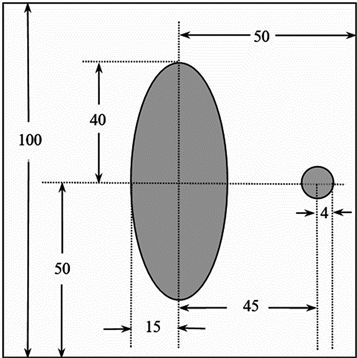Figure 1. Template map of the original data

$\left\{\begin{array}{l}Se=1-\frac{1}{size\left({I}_{1}^{\left(k\right)}\right)}\underset{\left(i,j\right)\in {I}_{1}^{\left(k\right)}}{\sum }|{I}^{\left(k\right)}\left(i,j\right)-{J}^{\left(k\right)}\left(i,j\right)|,\hfill \\ Sp=1-\frac{1}{size\left({I}_{0}^{\left(k\right)}\right)}\underset{\left(i,j\right)\in {I}_{0}^{\left(k\right)}}{\sum }|{I}^{\left(k\right)}\left(i,j\right)-{J}^{\left(k\right)}\left(i,j\right)|\hfill \\ Youden=Se+Sp-1.\hfill \end{array},$ (8)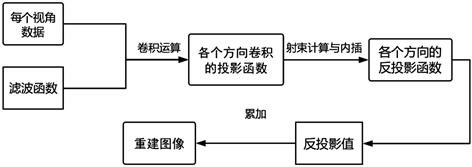Figure 2. Reconstruction flow chart based on convolution back projection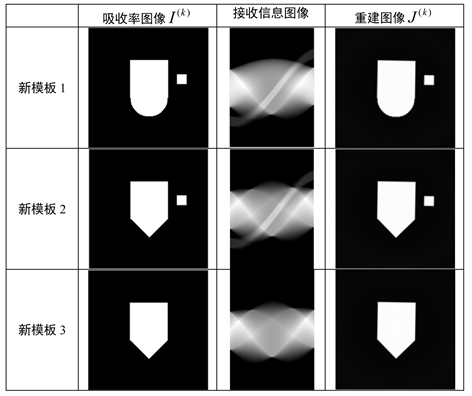Figure 3. Reception information and reconstruction effect of new templates. The first column is absorptivity matrix gray image; the second column is receiving information gray image; and the third column is the reconstructed imageTable 1. Reconstruction effect of original template and three new templates

5. 结语Table 2. Youden index of reconstruction effect of original template and new templates under different noise intensity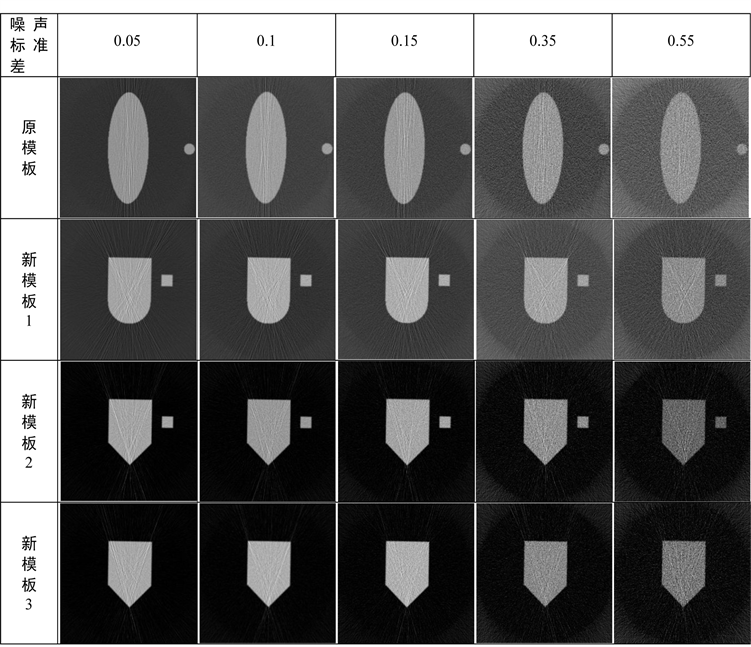Figure 4. Reconstruction effect diagram of original template and new templates under different noise intensity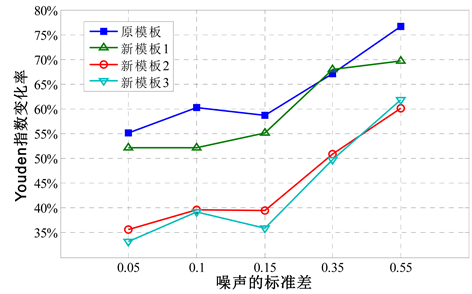Figure 5. The change rate of Youden index of original template and the three new templates with increasing noise intensity

NOTES

*通讯作者。

  李想. CT图像的应用研究[D]: [硕士学位论文]. 哈尔滨: 哈尔滨工程大学, 2004.  Sidky, E.Y., Zou, Y. and Pan, X. (2005) Volume Image Reconstruction from a Straight-Line Source Trajectory. Nuclear Science Symposium Conference Record, Fajardo, 23-29 October 2005, 2441-2444.  Katsevich, A. (2002) Theoretically Exact Filtered Backprojection-Type Inversion Algorithm for Spiral CT. Siam Journal on Applied Mathematics, 62, 2012-2026. https://doi.org/10.1137/S0036139901387186  郭威. CT不完全投影数据重建算法研究[D]: [博士学位论文]. 长春: 吉林大学, 2011.  莫华, 龙莉玲. X-CT图像重建的卷积反投影图解法[J]. 中国医学物理学杂志, 1999, 16(3): 143-145.  Wang, F., Wang, L. and Zou, R.B. (2014) Multifractal Detrended Moving Average Analysis for Texture Representation. Chaos: An Interdisciplinary Journal of Nonlinear Science, 24, Article ID: 033127.  王晓鹏, 王明泉, 侯慧玲. 基于R-L-MS-L滤波函数的CT图像重建[J]. 电视技术, 2014, 38(7): 26-28.  庄天戈. CT原理与算法[M]. 上海: 上海交通大学出版社, 1992.  刘云云. 基于纹理子空间成分的活动轮廓模型的图像分割[D]: [硕士学位论文]. 大连: 大连理工大学, 2009.  李保磊, 张耀军. 基于对称投影的2D-CT系统投影旋转中心自动确定方法[C]//全国射线数字成像与CT新技术研讨会论文集. 上海.  王金平. 平面Radon变换的反演公式[J]. CT理论与应用研究, 2000, 9(1): 8-11.  杨志伟, 傅炳南. 发热出疹性疾病的临床诊断及其特异度和敏感度的分析[J]. 中国疫苗和免疫, 1999(2): 76-79.  李雪迎. 诊断能力评价的统计学方法——准确度与约登指数[J]. 中国介入心脏病学杂志, 2011, 19(4): 213-213.  赵勇, 李品江. CT噪声和伪影对成像质量的影响及控制[J]. 中华放射学杂志, 1997(1): 56-58.  王卫江. 实时高斯噪声产生方法[J]. 仪器仪表学报, 2006, 27(11): 1523-1525.